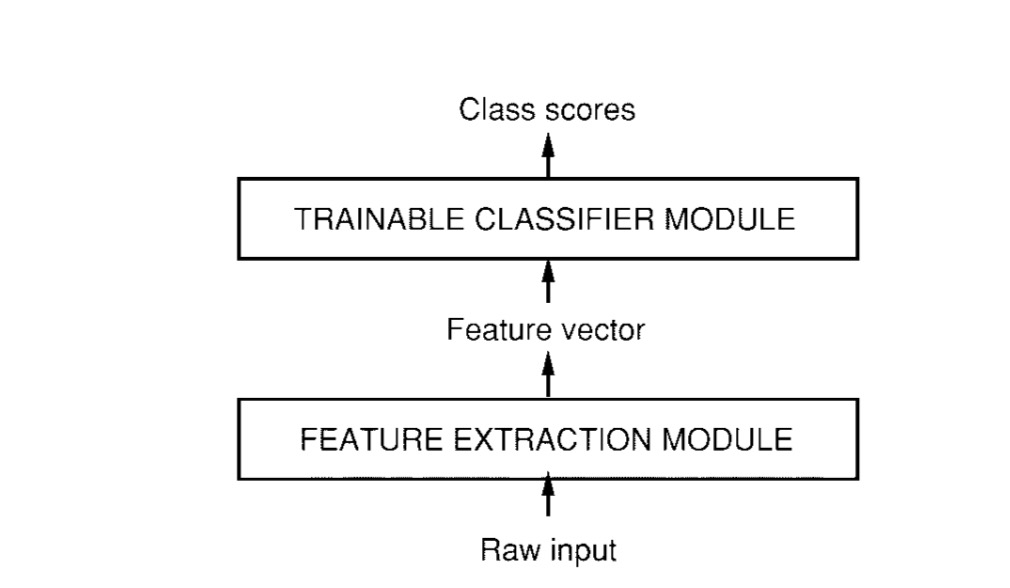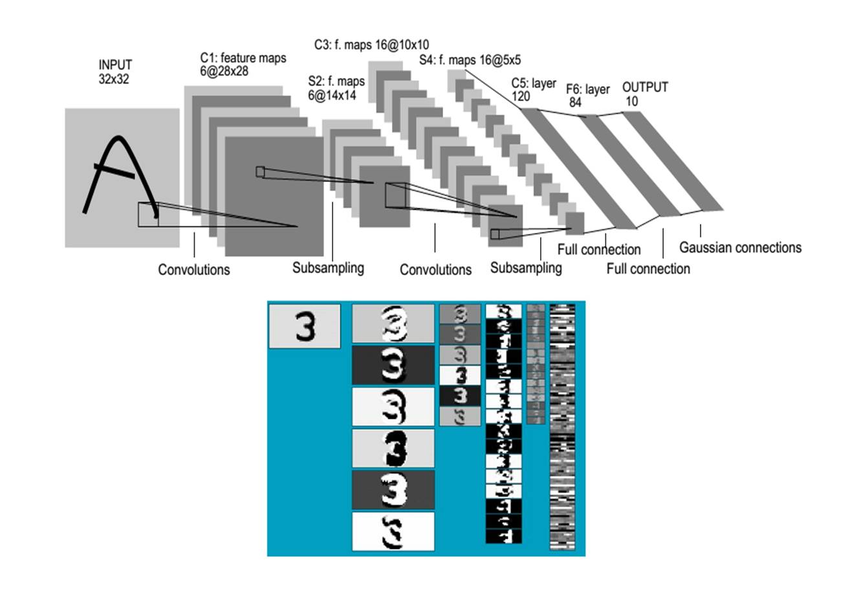# LeNet

## 项目简介

1994 年深度学习三巨头之一的 Yan LeCun 提出了 LeNet 神经网络，这是最早的卷积神经网络。1998 年 Yan LeCun 在论文 “Gradient-Based Learning Applied to Document Recognition” 中将这种卷积神经网络命名为 “LeNet-5”。LeNet-5 表明更好的模式识别系统可以建立在自动的学习上，更少的依赖手动设计的启发式模型。以字符识别为例，LeNet 表明手动选择的特征可以被更先进的直接在像素操作的学习机器取代。最早期的时候认为原始的数据非常多样并且丰富让模式识别被不能完全依靠手工建立一个准确的模式识别系统。所以，大部分的模式识别系统建立在自动学习技术和手工选择算法的结合。模式识别系统包含两个分开主要的模块，如图所示：Gradient-Based Learning Applied to Document Recognition
http://ieeexplore.ieee.org/stamp/stamp.jsp?tp=&arnumber=726791

00726791

Architecture of LeNet-5 (Convolutional Neural Networks) for digit recognition## 数据处理

TensorFlow 卷积神经网络手写数字识别数据集介绍

TensorFlow 卷积神经网络手写数字识别数据集介绍

## 模型实现

models/research/slim/nets/lenet.py
https://github.com/tensorflow/models/blob/master/research/slim/nets/lenet.py

``````import tensorflow as tf

slim = tf.contrib.slim

def lenet(images, num_classes=10, is_training=False,
dropout_keep_prob=0.5,
prediction_fn=slim.softmax,
scope='LeNet'):
end_points = {}
with tf.variable_scope(scope, 'LeNet', [images]):
net = end_points['conv1'] = slim.conv2d(images, 32, [5, 5], scope='conv1')
net = end_points['pool1'] = slim.max_pool2d(net, [2, 2], 2, scope='pool1')
net = end_points['conv2'] = slim.conv2d(net, 64, [5, 5], scope='conv2')
net = end_points['pool2'] = slim.max_pool2d(net, [2, 2], 2, scope='pool2')
net = slim.flatten(net)
end_points['Flatten'] = net

net = end_points['fc3'] = slim.fully_connected(net, 1024, scope='fc3')
if not num_classes:
return net, end_points
net = end_points['dropout3'] = slim.dropout(
net, dropout_keep_prob, is_training=is_training, scope='dropout3')
logits = end_points['Logits'] = slim.fully_connected(
net, num_classes, activation_fn=None, scope='fc4')

end_points['Predictions'] = prediction_fn(logits, scope='Predictions')

return logits, end_points
lenet.default_image_size = 28

def lenet_arg_scope(weight_decay=0.0):
"""Defines the default lenet argument scope.
Args:
weight_decay: The weight decay to use for regularizing the model.
Returns:
An `arg_scope` to use for the inception v3 model.
"""
with slim.arg_scope(
[slim.conv2d, slim.fully_connected],
weights_regularizer=slim.l2_regularizer(weight_decay),
weights_initializer=tf.truncated_normal_initializer(stddev=0.1),
activation_fn=tf.nn.relu) as sc:
return sc

``````